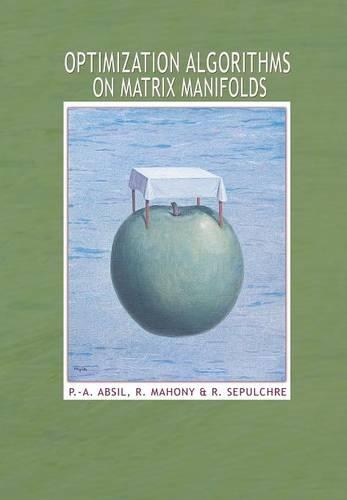•# Optimization Algorithms on Matrix Manifolds (Hardback)

(author), (author), (author)
£65.00
Hardback 240 Pages / Published: 18/01/2008
• We can order this

Usually dispatched within 3 weeks

Many problems in the sciences and engineering can be rephrased as optimization problems on matrix search spaces endowed with a so-called manifold structure. This book shows how to exploit the special structure of such problems to develop efficient numerical algorithms. It places careful emphasis on both the numerical formulation of the algorithm and its differential geometric abstraction--illustrating how good algorithms draw equally from the insights of differential geometry, optimization, and numerical analysis. Two more theoretical chapters provide readers with the background in differential geometry necessary to algorithmic development. In the other chapters, several well-known optimization methods such as steepest descent and conjugate gradients are generalized to abstract manifolds. The book provides a generic development of each of these methods, building upon the material of the geometric chapters. It then guides readers through the calculations that turn these geometrically formulated methods into concrete numerical algorithms. The state-of-the-art algorithms given as examples are competitive with the best existing algorithms for a selection of eigenspace problems in numerical linear algebra. Optimization Algorithms on Matrix Manifolds offers techniques with broad applications in linear algebra, signal processing, data mining, computer vision, and statistical analysis. It can serve as a graduate-level textbook and will be of interest to applied mathematicians, engineers, and computer scientists.

Publisher: Princeton University Press
ISBN: 9780691132983
Number of pages: 240
Weight: 454 g
Dimensions: 235 x 152 x 20 mm